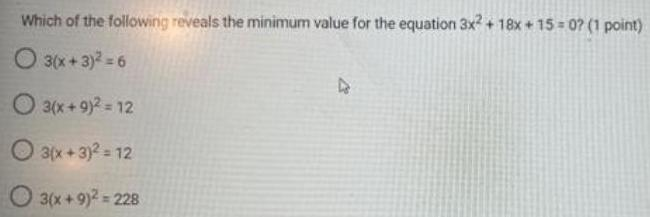Question:

# Which of the following reveals the minimum value for the

Last updated: 8/14/2022Which of the following reveals the minimum value for the equation 3x2 + 18x+15=0? 3(x+3)² = 6 3(x+9)² = 12 3(x+3)² = 12 3(x+9)² = 228